0
1060

# SSC CHSL Data Interpretation Questions:

Download Top-20 Data Interpretation questions for SSC CHSL exam. Most important  Data Interpretation Questions based on asked questions in previous exam papers for SSC CHSL.

InstructionsDirections :The pie chart, given here, represents the number of valid votes obtained by four students who contested election for school leadership. The total number of valid votes polled was 720. Observe the chart and answer the questions based on it.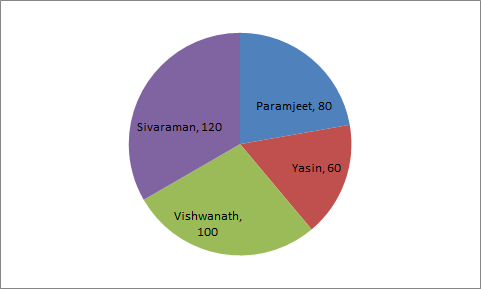Number represented in pie-chart are in degrees.

Question 1: What was the winner ?

a) Sivaraman

b) Paramjeet

c) Yasin

d) Vishwanath

InstructionsDirections : The following graph shows the production of cotton bales of 100 kg each in lakhs by different states A, B, C, D and E over the years. Study the graph and answer the following Questions.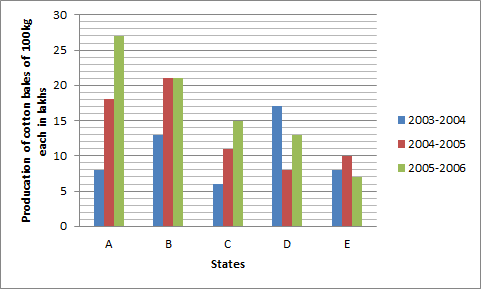Question 2: In which State(s) is there a steady increase in the production of cotton during the given period?

a) A and B

b) B and D

c) A and C

d) D and E

Question 3: How many kg of cotton was produced by State C during the given period?

a) 32,00,00,000 kg

b) 42,50,00,000 kg

c) 33,00,00,000 kg

d) 35,00,00,000 kg

Question 4: The number of States for which the production of cotton in 2005-2006 is less than or equal to the preceding year is

a) 3

b) 2

c) 1

d) There is no such States

Instructions

Directions : Population of the five adjacent areas of a town, in the year of 2020, are represented in the following Pie-chart. The ratio of the numbers of males to that of females in these areas are states in the table below. The total of the population in all the five areas is 72 lakh. Study the Pie-chart and the table and then answer the questions.

Ratio of number of males(M) to females(F)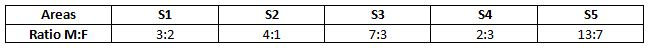Question 5: 12 lakh in the population of the area

a) S1

b) S3

c) S5

d) S4

Question 6: The number of males in the areas S1 and S4 together is

a) 13.8 lakh

b) 8.2 lakh

c) 16.2 lakh

d) 15.8 lakh

Instructions

Study the following Histogram and answer the following questions.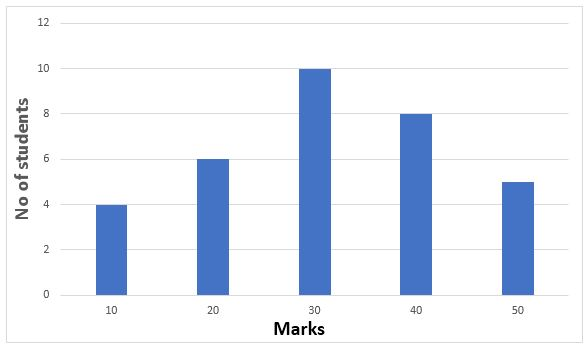Question 7: The total number of students involved in the data is

a) 33

b) 32

c) 43

d) 42

Question 8: The maximum number of students got the marks in the interval of

a) 10-20

b) 20-30

c) 30-40

d) 40-50

Instructions

The pie-chart shows the proposed outlay for different sectors during a Five-Year plan of Government of India. Total outlay is Rs. 40,000 crores. By reading the pie-chart answer the following three questions.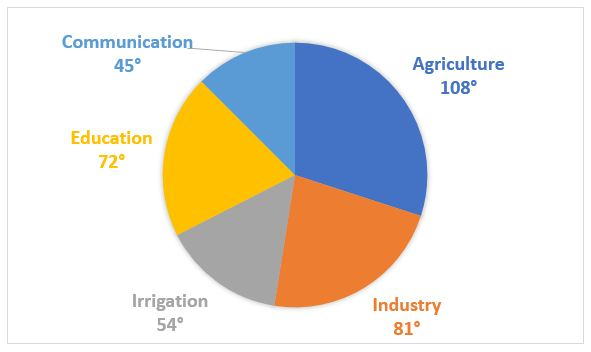Question 9: If the proposed outlay of Irrigation is x% of the proposed outlay of Agriculture, then x is equal to

a) 50%

b) 15%

c) 25%

d) 75%

Question 10: What is the ratio between the proposed outlay of Irrigation and Communication?

a) 9 : 8

b) 3 : 2

c) 9 : 5

d) 6 : 5

Instructions

Study the following bar diagram carefully and answer the following questions.

The number of the production of electronic items (TVs and LCDs) in a factory during the period from 2009 to 2013.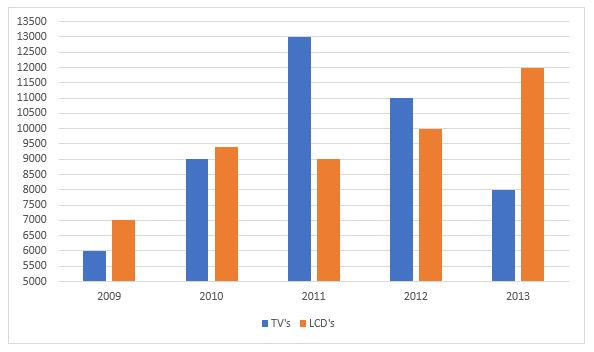Question 11: The total number of products of electronic items is maximum in the year

a) 2009

b) 2010

c) 2011

d) 2013

Question 12: The ratio of production of LCDs in the year 2011 and 2013 is

a) 3 : 4

b) 4 : 3

c) 2 : 3

d) 1 : 4

Question 13: The difference between averages of production of TVs and LCDs from 2009 to 2012 is

a) 600

b) 700

c) 800

d) 900

Question 14: The ratio of production of TVs in the years 2009 and 2010 is

a) 7 : 6

b) 6 : 7

c) 2 : 3

d) 3 : 2

Instructions

The following pie-chart shows the sources of funds (InRs. crores) to be collected by the National Highways Authority of India (NHAI) for its Phase II projects. Study the pie-chart and answer the following Three questions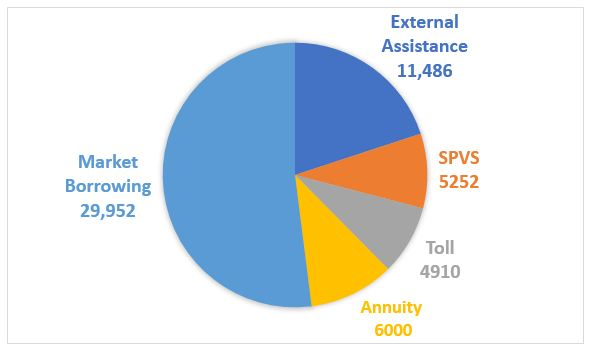Question 15: If the toll is to be collected through an outsourced agency by allowing a maximum 10% commission, how much amount should be permitted to be collected by the outsourced agency, so that the project is supported with Rs. 4,910 crores ?

a) Rs. 6,213 crores

b) Rs. 5,827 crores

c) Rs. 5,401 crores

d) Rs. 5,316 crores

Question 16: If NHAI could receive a total of Rs. 9,695 crores as External Assistance, by what percent (approximately) should it increase the Market Borrowing to arrange for the shortage of funds ?

a) 4.5%

b) 7.5%

c) 6%

d) 8%

Instructions

Study the following frequency polygon and answer the questions. Given a line graph showing the number of students passed in Higher Secondary Examination in a school over the years 2008 to 2013.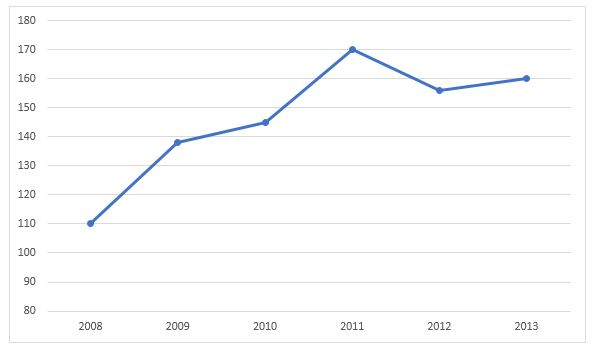Question 17: The average of passed students in the years 2008, 2009, 2012 approximately is

a) 134.32

b) 134.41

c) 134.56

d) 134.67

Question 18: The increase in percentage of passed students from 2008 to 2011 approximately is

a) 55%

b) 50.5%

c) 54.5%

d) 53.05%

Question 19: The decrease in percentage of passed students from 2011 to 2012 approximately is

a) 8.25%

b) 8.27%

c) 8.24%

d) 8.22%

Instructions

In the following questions, the graph shows the demand and production of different companies. Study the graph and answer the questions.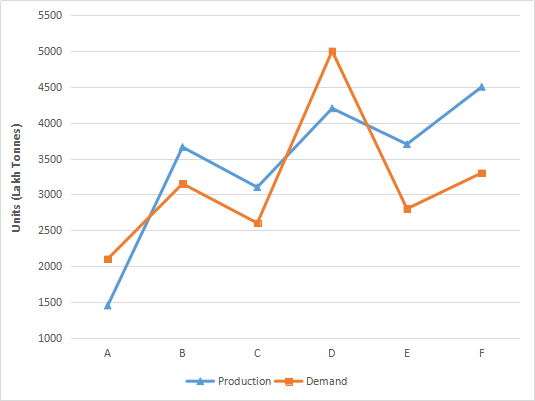Question 20: The demand of company E is approximately what percentage of the production of company F ?

a) 60%

b) 70%

c) 80%

d) 50%

Person having maximum no. of distribution will have max. no. of votes

Only in A and C there is a steady increment in production of cotton as in D and E , It is decresed and in B production is equal for two years. Hence answer will be C).

Production of C in year 2003-2004 = 6 per 100 kg in lakh
in year 2004-05 = 11 per 100 kg in lakh
in year 2005-06 = 15 per 100 kg in lakh
Total will be 32 per 100 kg in lakh or 320000000 kg

In B, production is same as its previous year whether in C , it is decreased.

Total no. of students involved in the data is 4 +6 +10+ 8+ 5 = 33.

Clearly, the maximum no. of 10 students got in the interval of 20-30 marks

Total outlay = 40,000 cr

Proposed outlay for irrigation = $\frac{54}{360}$ * 40,000 = 6,000 cr

Proposed outlay for agriculture = $\frac{108}{360}$ * 40,000 = 12,000 cr

Now, outlay for irrigation is x% of that of agriculture.

=> $\frac{6000}{12000}$ * 100 = 50%

=> x = 50

(SHORTCUT)

Degree outlay for irrigation = 54 and for agriculture = 108

Required % = $\frac{54}{108}$ * 100 = 50%

=> x = 50

Degree of proposed outlay for irrigation = 54

Degree of proposed outlay for communication = 45

Required ratio = 54/45 = 6 : 5

Total no. of electronic items in :

2009 = 6000 + 7000 = 13000

2010 = 9000 + 9400 = 18400

2011 = 13000 + 9000 = 22000 [MAX]

2012 = 11000 + 10000 = 21000

2013 = 8000 + 12000 = 20000

=> Total production is maximum in 2011

Total production of LCD’s in 2011 = 9000

Total production of LCD’s in 2013 = 12000

Require ratio = 9000/12000 = 3 : 4

Total no. of TV’s produced from 2009 to 2012 = 6000 + 9000 + 13000 + 11000

=> 39,000

Average production of TV’s over these four years = 39000/4 = 9750

Total no. of LCD’s produced from 2009 to 2012 = 7000 + 9400 + 9000 + 10000 = 35,400

Average production of LCD’s over these four years = 35400/4 = 8850

=> Required Difference = 9750 – 8850 = 900

No. of TV’s produced in 2009 = 6000

No. of TV’s produced in 2010 = 9000

=> Required ratio = 6000/9000 = 2 : 3

Amount required to support the project = 4910 crore

Maximum compensation = 10%

=> 10% of 4910 cr = $\frac{10}{100} * 4910 cr = 491 cr Amount permitted = 4910 cr + 491 cr = 5401 cr 16) Answer (C) Shortage of funds arranged through External Assistance = 11486 – 9695 = 1791 crores => Increase required in market borrowing = Rs. 1791 crore % increase required =$\frac{1791}{29952}$* 100 = 5.98% = ~6% 17) Answer (D) No. of passed students in 2008 = 110 No. of passed students in 2009 = 138 No. of passed students in 2012 = 156 => Total no. of students passed in 2008, 2009 & 2012 = 110+138+156 = 404 => Average students passed over the three years = 404/3 = 134.67 18) Answer (C) No. of students passed in 2008 = 110 No. of passed students in 2011 = 170 => % increase =$\frac{170-110}{110}$* 100 = 54.54% = ~54.5% 19) Answer (C) No. of students passed in 2011 = 170 No. of passed students in 2012 = 156 => % decrease =$\frac{170-156}{170} * 100 = 8.235 \%\$

= ~8.24%